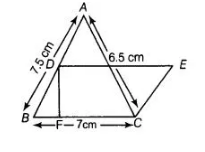# In figure, ΔABC has sides AB = 7.5 cm,`
Question:

In figure, ΔABC has sides AB = 7.5 cm, AC = 6.5 cm and BC = 7 cm. On base BC a parallelogram DBCE of same area as that of ΔABC is constructed.

Find the height OF of the parallelogram.Thinking process

(i) First, determine the area of AABC by using Heron’s formula i.e.,

$\sqrt{s(s-a)(s-b)(s-c)}$

(ii) Second, determine the area of parallelogram BCED by using the formula Base x Height.

(iii) Further, equate the area of triangle and area of parallelogram, to get the height of the parallelogram.

Solution:

Now, first determine the area of $\triangle A B C$.

The sides of a triangle are

$A B=\mathrm{a}=7.5 \mathrm{~cm}, \mathrm{BC}=\mathrm{b}=7 \mathrm{~cm}$ and $C A=c=6.5 \mathrm{~cm}$

Now, semi-perimeter of a triangle,

$s=\frac{a+b+c}{2}=\frac{7.5+7+6.5}{2}=\frac{21}{2}=10.5 \mathrm{~cm}$

$\therefore$ Area of $\triangle A B C=\sqrt{s(s-a)(s-b)(s-c)}$ [by Heron's formula]

$=\sqrt{10.5(10.5-7.5)(10.5-7)(10.5-6.5)}$

$=\sqrt{10.5 \times 3 \times 3.5 \times 4}=\sqrt{441}=21 \mathrm{~cm}^{2}$  $\ldots$ (i)

Now, area of parallelogram $B C E D=$ Base $\times$ Height

$=B C \times D F=7 \times D F$ .....(ii)

According to the question,

Area of $\triangle A B C=$ Area of parallelogram $B C E D$

$\Rightarrow$ $21=7 \times D F$ [from Eqs. (i) and (ii)]

$\Rightarrow$ $D F=\frac{21}{4}=3 \mathrm{~cm}$

Hence, the height of parallelogram is $3 \mathrm{~cm}$.